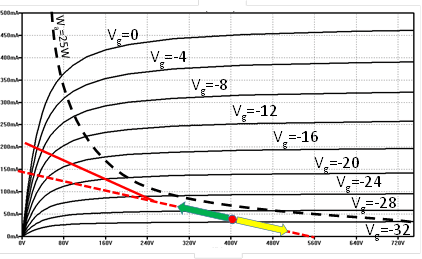In a push pull output transformer, the impedance rating is referred to the whole impedance seen from one end to the other end of the primary. Since two vacuum tubes simultaneously use the transformer, each tube sees half impedance of the transformer. For instance, when using a push pull transformer with an impedance of 7.6K Ohm, each anode sees an impedance of 3.8K Ohm. The loadline, in this case, has to be also drawn considering half the transformer impedance.

The method for drawing the loadline has already been discussed in Section 4.1.2. However, at a closer look, drawing the push-pull loadline in class AB require additional considerations to be made.

We just said that the impedance seen by each anode is half that of the end-to-end impedance of the transformer primary. This is true only when both vacuum tubes are conducting simultaneously, for instance, when both vacuum tubes operate in class A. However, class AB operation is generally used In a push-pull power stage. In a class AB amplifier, as discussed in Sections 3.5 and 4.1.5, one vacuum tube might quit conducting while the other is fully active, and vice versa. When one of the two vacuum tubes does not conduct, just half transformer is used. In this case, the impedance seen by the anode is not half than that of the entire transformer. In fact, we already said that the impedance of the transformer goes with the square of the ratio between the number of turns, of the primary and the secondary of the transformer. When we use half transformer, we use half turns of the transformer primary and the impedance seen at the anode is one fourth of the entire transformer impedance. This can be verified using the equations discussed in Section 4.1.2.

Figure 24 clarifies these aspects. When the two vacuum tubes amplify each, a signal inverted with respect to the other, their operating points move in opposite directions along the loadline, starting from the quiescent operating point. The green and the yellow arrow represent the simultaneous position of the two vacuum tubes along the loadline. Suppose the signal amplified by the yellow vacuum tube is such that it reaches the bottom of the graph and quits conducting. The green vacuum tube, at this point, sees a different impedance at its anode and the loadline slope changes accordingly. From this point on, the green arrow follows the solid red line, rather than the dashed line corresponding to the continuation of the previous loadline. When the signal being amplified is such that the yellow vacuum tube starts conducting again, the green arrow will be back at the position where the impedance first changed. At this point, the green vacuum tube sees the original impedance again, and continues along the solid red line. Note, of course, that the impedance change is not abrupt as depicted in the figure. When the yellow vacuum tube is in the process of quitting conduction, the impedance seen by the other tube changes gradually. Try also the loadline calculator for vacuum tubes and experiment with various settings.Figure 24: Loadline in a class AB push-pull amplifier.In a push-pull transformer, operating in class AB, the loadline is not a straight line. While both vacuum tubes are conducting, the impedance seen at the anode is half than that of the entire transformer. When one of the two vacuum tubes quits conducting, just half transformer is used, and given that the impedance goes with the square of the turn ratio, the impedance will be one fourth of the entire transformer impedance. In the picture, when the yellow arrow, representing one power vacuum tube, reaches the bottom, the green arrow, representing the other vacuum tube, continues following the solid red line, rather than the dashed line.